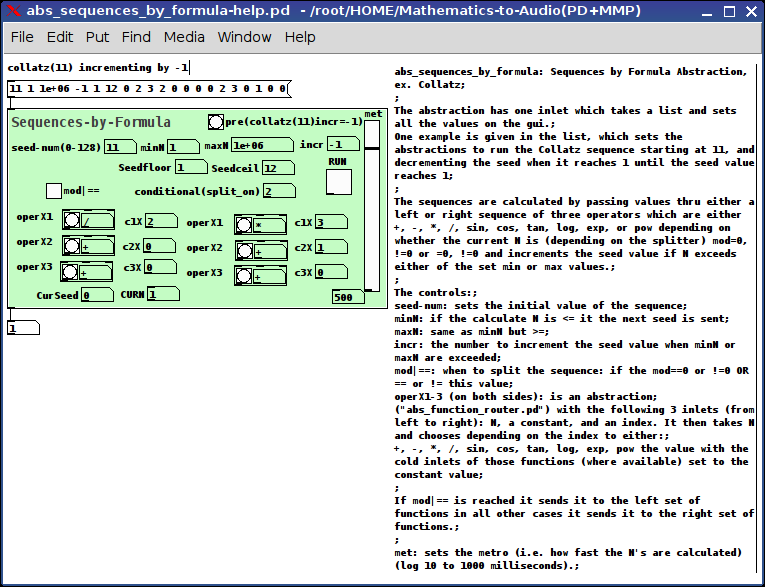abs_sequences_by_formula: Sequences by Formula Abstraction, ex. CollatzReally I was just curious to see if I could do this.

As to practical purpose: unknown (tho I Do think it's cool to stack sequences with a function router and might come in handy for those folks pursuing Sequencers)

The abstraction has one inlet which takes a list and sets all the values on the gui.
One example is given in the list, which sets the abstraction to run the Collatz sequence starting at 11, and decrementing the seed when it reaches 1 until the seed value reaches 1.

The sequences are calculated by passing values thru either a left or right sequence of three operators which are either +,-,*,/,sin,cos,tan,log,exp, or pow depending on whether the current N is (depending on the splitter) mod=0,!=0 or =0,!=0 and increments the seed value if N exceeds either of the set min or max values.

The controls:
seed-num: sets the initial value of the sequence;
minN: if the calculate N is <= it the next seed is sent;
maxN: same as minN but >=;
incr: the number to increment the seed value when minN or maxN are exceeded;
mod|==: when to split the sequence: if the mod==0 or !=0 OR == or != this value;
operX1-3 (on both sides): is an abstraction ("abs_function_router.pd") with the following 3 inlets (from left to right): N, a constant, and an index. It then takes N and chooses depending on the index to either: +,-,*,/,sin,cos,tan,log,exp,pow the value with the cold inlets of those functions (where available) set to the constant value;

If mod|== is reached it sends it to the left set of functions in all other cases it sends it to the right set of functions.

met: sets the metro (i.e. how fast the N's are calculated) (log 10 to 1000 milliseconds).

Footnote:

My intention here was to make a tool available which might allow us as pd users to show non-pd users what we mean when we say "Mathematics is 'Musical'".

Ciao!.

Have fun. Let me know if you need any help.

Peace.
Scott